# Colormap Normalization¶

Objects that use colormaps by default linearly map the colors in the colormap from data values vmin to vmax. For example:

pcm = ax.pcolormesh(x, y, Z, vmin=-1., vmax=1., cmap='RdBu_r')


will map the data in Z linearly from -1 to +1, so Z=0 will give a color at the center of the colormap RdBu_r (white in this case).

Matplotlib does this mapping in two steps, with a normalization from the input data to [0, 1] occurring first, and then mapping onto the indices in the colormap. Normalizations are classes defined in the matplotlib.colors() module. The default, linear normalization is matplotlib.colors.Normalize().

Artists that map data to color pass the arguments vmin and vmax to construct a matplotlib.colors.Normalize() instance, then call it:

In : import matplotlib as mpl

In : norm = mpl.colors.Normalize(vmin=-1.,vmax=1.)

In : norm(0.)
Out: 0.5


However, there are sometimes cases where it is useful to map data to colormaps in a non-linear fashion.

## Logarithmic¶

One of the most common transformations is to plot data by taking its logarithm (to the base-10). This transformation is useful to display changes across disparate scales. Using colors.LogNorm normalizes the data via $$log_{10}$$. In the example below, there are two bumps, one much smaller than the other. Using colors.LogNorm, the shape and location of each bump can clearly be seen:

import numpy as np
import matplotlib.pyplot as plt
import matplotlib.colors as colors
import matplotlib.cbook as cbook

N = 100
X, Y = np.mgrid[-3:3:complex(0, N), -2:2:complex(0, N)]

# A low hump with a spike coming out of the top right.  Needs to have
# z/colour axis on a log scale so we see both hump and spike.  linear
# scale only shows the spike.
Z1 = np.exp(-(X)**2 - (Y)**2)
Z2 = np.exp(-(X * 10)**2 - (Y * 10)**2)
Z = Z1 + 50 * Z2

fig, ax = plt.subplots(2, 1)

pcm = ax.pcolor(X, Y, Z,
norm=colors.LogNorm(vmin=Z.min(), vmax=Z.max()),
cmap='PuBu_r')
fig.colorbar(pcm, ax=ax, extend='max')

pcm = ax.pcolor(X, Y, Z, cmap='PuBu_r')
fig.colorbar(pcm, ax=ax, extend='max')
plt.show()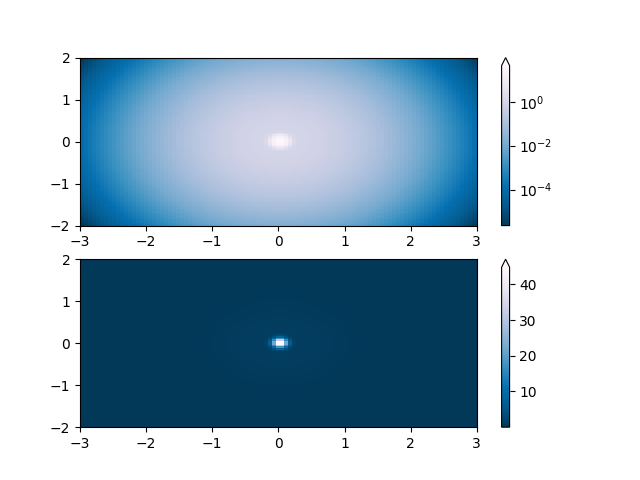## Symmetric logarithmic¶

Similarly, it sometimes happens that there is data that is positive and negative, but we would still like a logarithmic scaling applied to both. In this case, the negative numbers are also scaled logarithmically, and mapped to smaller numbers; e.g., if vmin=-vmax, then they the negative numbers are mapped from 0 to 0.5 and the positive from 0.5 to 1.

Since the logarithm of values close to zero tends toward infinity, a small range around zero needs to be mapped linearly. The parameter linthresh allows the user to specify the size of this range (-linthresh, linthresh). The size of this range in the colormap is set by linscale. When linscale == 1.0 (the default), the space used for the positive and negative halves of the linear range will be equal to one decade in the logarithmic range.

N = 100
X, Y = np.mgrid[-3:3:complex(0, N), -2:2:complex(0, N)]
Z1 = np.exp(-X**2 - Y**2)
Z2 = np.exp(-(X - 1)**2 - (Y - 1)**2)
Z = (Z1 - Z2) * 2

fig, ax = plt.subplots(2, 1)

pcm = ax.pcolormesh(X, Y, Z,
norm=colors.SymLogNorm(linthresh=0.03, linscale=0.03,
vmin=-1.0, vmax=1.0, base=10),
cmap='RdBu_r')
fig.colorbar(pcm, ax=ax, extend='both')

pcm = ax.pcolormesh(X, Y, Z, cmap='RdBu_r', vmin=-np.max(Z))
fig.colorbar(pcm, ax=ax, extend='both')
plt.show()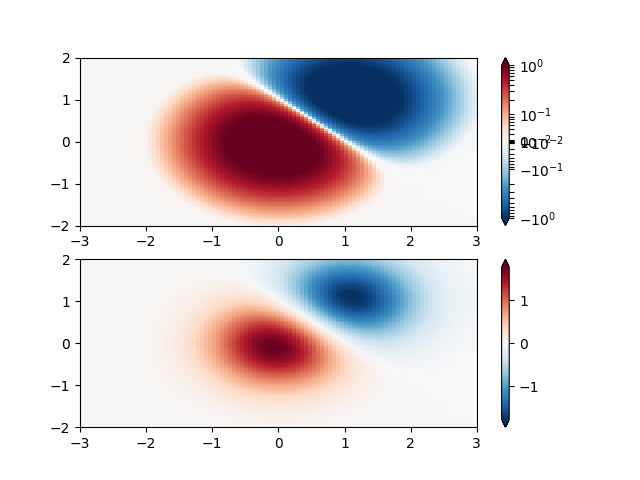## Power-law¶

Sometimes it is useful to remap the colors onto a power-law relationship (i.e. $$y=x^{\gamma}$$, where $$\gamma$$ is the power). For this we use the colors.PowerNorm. It takes as an argument gamma (gamma == 1.0 will just yield the default linear normalization):

Note

There should probably be a good reason for plotting the data using this type of transformation. Technical viewers are used to linear and logarithmic axes and data transformations. Power laws are less common, and viewers should explicitly be made aware that they have been used.

N = 100
X, Y = np.mgrid[0:3:complex(0, N), 0:2:complex(0, N)]
Z1 = (1 + np.sin(Y * 10.)) * X**(2.)

fig, ax = plt.subplots(2, 1)

pcm = ax.pcolormesh(X, Y, Z1, norm=colors.PowerNorm(gamma=0.5),
cmap='PuBu_r')
fig.colorbar(pcm, ax=ax, extend='max')

pcm = ax.pcolormesh(X, Y, Z1, cmap='PuBu_r')
fig.colorbar(pcm, ax=ax, extend='max')
plt.show()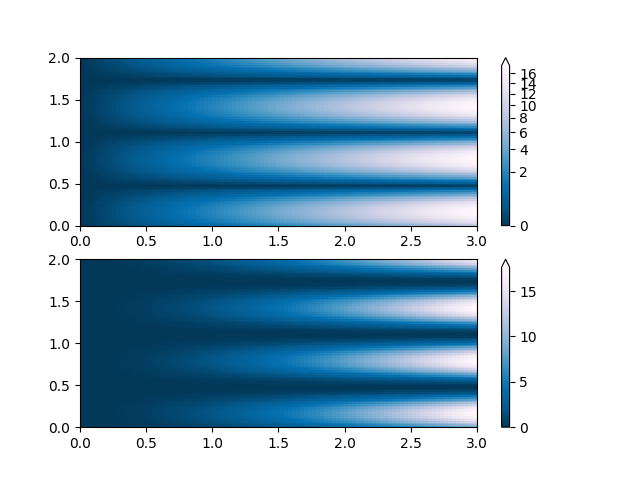## Discrete bounds¶

Another normalization that comes with Matplotlib is colors.BoundaryNorm. In addition to vmin and vmax, this takes as arguments boundaries between which data is to be mapped. The colors are then linearly distributed between these "bounds". For instance:

In : import matplotlib.colors as colors

In : bounds = np.array([-0.25, -0.125, 0, 0.5, 1])

In : norm = colors.BoundaryNorm(boundaries=bounds, ncolors=4)

In : print(norm([-0.2,-0.15,-0.02, 0.3, 0.8, 0.99]))
[0 0 1 2 3 3]


Note: Unlike the other norms, this norm returns values from 0 to ncolors-1.

N = 100
X, Y = np.mgrid[-3:3:complex(0, N), -2:2:complex(0, N)]
Z1 = np.exp(-X**2 - Y**2)
Z2 = np.exp(-(X - 1)**2 - (Y - 1)**2)
Z = (Z1 - Z2) * 2

fig, ax = plt.subplots(3, 1, figsize=(8, 8))
ax = ax.flatten()
# even bounds gives a contour-like effect
bounds = np.linspace(-1, 1, 10)
norm = colors.BoundaryNorm(boundaries=bounds, ncolors=256)
pcm = ax.pcolormesh(X, Y, Z,
norm=norm,
cmap='RdBu_r')
fig.colorbar(pcm, ax=ax, extend='both', orientation='vertical')

# uneven bounds changes the colormapping:
bounds = np.array([-0.25, -0.125, 0, 0.5, 1])
norm = colors.BoundaryNorm(boundaries=bounds, ncolors=256)
pcm = ax.pcolormesh(X, Y, Z, norm=norm, cmap='RdBu_r')
fig.colorbar(pcm, ax=ax, extend='both', orientation='vertical')

pcm = ax.pcolormesh(X, Y, Z, cmap='RdBu_r', vmin=-np.max(Z))
fig.colorbar(pcm, ax=ax, extend='both', orientation='vertical')
plt.show()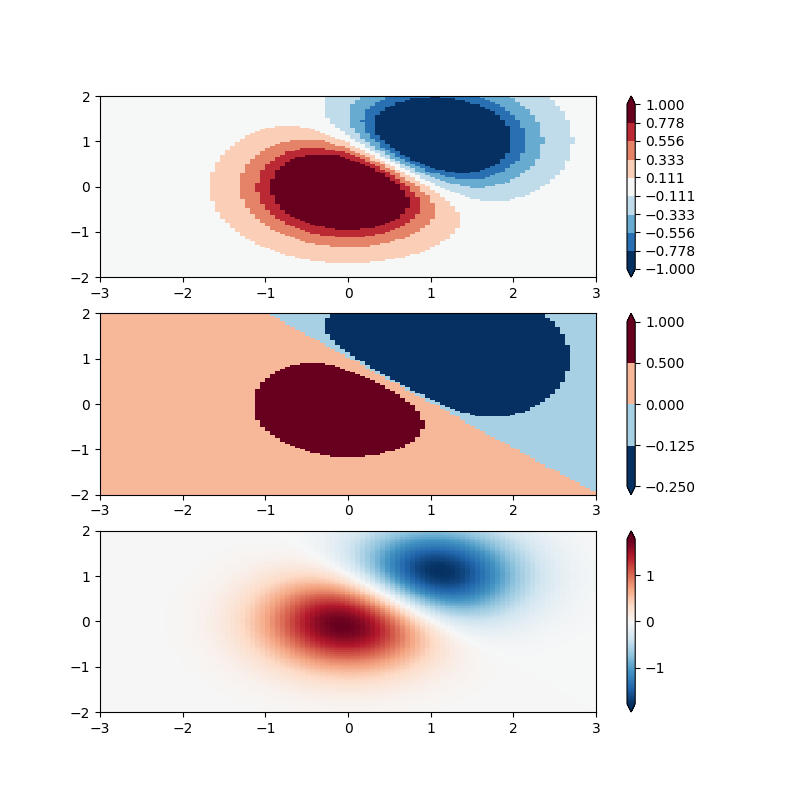## TwoSlopeNorm: Different mapping on either side of a center¶

Sometimes we want to have a different colormap on either side of a conceptual center point, and we want those two colormaps to have different linear scales. An example is a topographic map where the land and ocean have a center at zero, but land typically has a greater elevation range than the water has depth range, and they are often represented by a different colormap.

filename = cbook.get_sample_data('topobathy.npz', asfileobj=False)
with np.load(filename) as dem:
topo = dem['topo']
longitude = dem['longitude']
latitude = dem['latitude']

fig, ax = plt.subplots()
# make a colormap that has land and ocean clearly delineated and of the
# same length (256 + 256)
colors_undersea = plt.cm.terrain(np.linspace(0, 0.17, 256))
colors_land = plt.cm.terrain(np.linspace(0.25, 1, 256))
all_colors = np.vstack((colors_undersea, colors_land))
terrain_map = colors.LinearSegmentedColormap.from_list('terrain_map',
all_colors)

# make the norm:  Note the center is offset so that the land has more
# dynamic range:
divnorm = colors.TwoSlopeNorm(vmin=-500., vcenter=0, vmax=4000)

pcm = ax.pcolormesh(longitude, latitude, topo, rasterized=True, norm=divnorm,
cmap=terrain_map,)
# Simple geographic plot, set aspect ratio beecause distance between lines of
# longitude depends on latitude.
ax.set_aspect(1 / np.cos(np.deg2rad(49)))
fig.colorbar(pcm, shrink=0.6)
plt.show()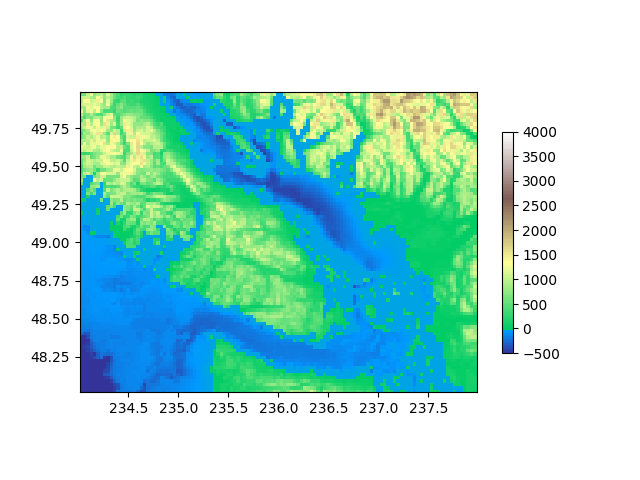## Custom normalization: Manually implement two linear ranges¶

The TwoSlopeNorm described above makes a useful example for defining your own norm.

class MidpointNormalize(colors.Normalize):
def __init__(self, vmin=None, vmax=None, vcenter=None, clip=False):
self.vcenter = vcenter
colors.Normalize.__init__(self, vmin, vmax, clip)

def __call__(self, value, clip=None):
# I'm ignoring masked values and all kinds of edge cases to make a
# simple example...
x, y = [self.vmin, self.vcenter, self.vmax], [0, 0.5, 1]
return np.ma.masked_array(np.interp(value, x, y))

fig, ax = plt.subplots()
midnorm = MidpointNormalize(vmin=-500., vcenter=0, vmax=4000)

pcm = ax.pcolormesh(longitude, latitude, topo, rasterized=True, norm=midnorm,
cmap=terrain_map)
ax.set_aspect(1 / np.cos(np.deg2rad(49)))
fig.colorbar(pcm, shrink=0.6, extend='both')
plt.show()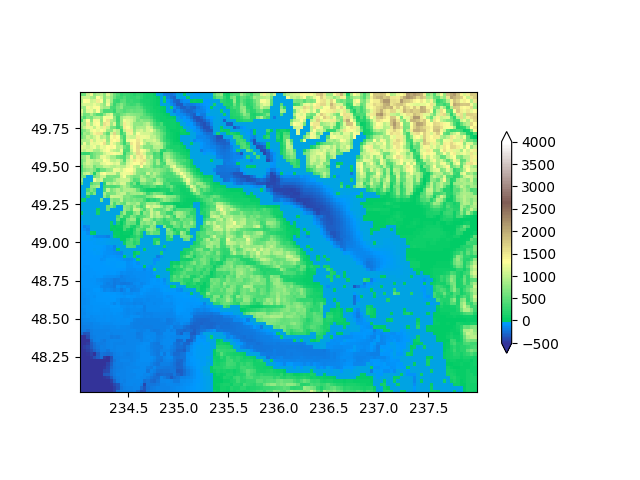Keywords: matplotlib code example, codex, python plot, pyplot Gallery generated by Sphinx-Gallery